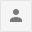Hi!  I need to create a graphic that shows in real time RGB from a video. I know that I have to capture a frame and plot 3 channels in a graphic and in the axis x plot time. But I am having a error. Could help me? Or do you have any ideas that how can I write this code?

I am trying to develop the code below but I am having a error:

```import cv2
import matplotlib.pyplot as plt
import numpy as np
from matplotlib.animation import FuncAnimation

capture = cv2.VideoCapture(0)

fig = plt.figure()

while True:
cv2.imshow('Frame', frame)

if not ret:
break

def animate(i):

b, g, r = cv2.split(frame)
xs = []
ys = []
for line in b:
if len(line) > 1:
x, y = np.prod(frame.shape)
xs.append(x)
ys.append(y)
ax1.clear()
ax1.plot(xs, ys)

ani = FuncAnimation(fig, animate, interval=1000)
plt.show()

keyval = cv2.waitKey(1) & 0xFF

if keyval == ord('q'):
break

capture.release()
cv2.destroyAllWindows()```Sep 16, 2020 in Python 398 views
Hey, @Leonardo,

Kindly post your error logs here. You have just given the code, kindly paste the error so that we can resolve your isue ASAP.

Thank you for your answer! Let me explain better what I need. While the webcam is opened, I want to show a graph that has at the axis 'x' time in seconds and at the axis 'y' pixel value. Three channels, three lines showing the variation of the channel beyond the time. The code that I wrote above I am trying to do that. Passing an empty list while the loop while is running. But I don't know whether my logic is right or not

The error that I am getting is:

```'numpy.ndarray has no attribute split'.
```

Hi,

Check your function animate(). You have used cv2.split() inside your function. But inside the function how you passed the value of the frame?

## Problem in real time matplotlib plotting

Hi Sucheta, The error I can see in ...READ MORE

## How to scrape time-series chart data from poocoin.app with Python?

In order to ensure it works, one ...READ MORE

## How can I find out the index of an element from row and column in Python?

You probably want to use np.ravel_multi_index: [code] import numpy ...READ MORE

+1 vote

## How to create plots using python matplotlib in IPython notebook?

I think you should try: I used %matplotlib inline in ...READ MORE

+1 vote

## How to handle Real-Time Matplotlib Plotting

To draw a continuous set of random ...READ MORE